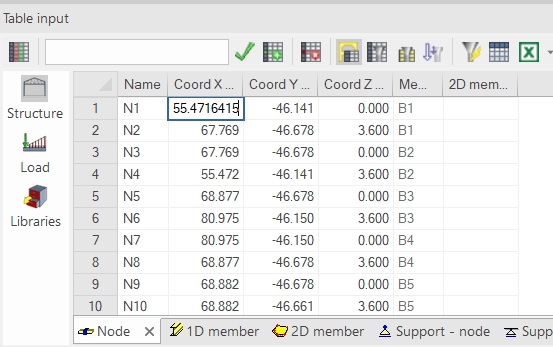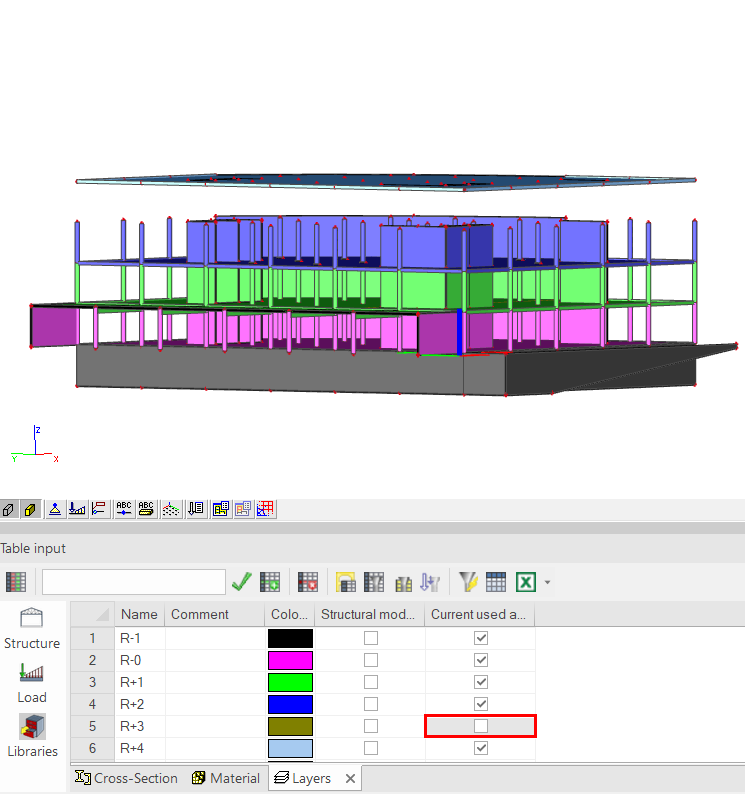# Table input tricks and tipsThe table input toolbar is a user interface component that helps you create and maintain geometry and loads in a project.

Following tips and tricks can help you save a lot of time and effort:

• rename several items (nodes, elements, supports, loads, …) at once;
• round coordinates to a certain number of digits;
• modify coordinates with simple mathematical formulas;
• select objects based on certain properties;
• quickly show or hide specific layers.

## Renaming several items at once

Table input allows you to rename an item. You can apply this to all data (nodes, elements, supports, loads, …). When multiple names are selected in the table grid they are all renamed and also automatically renumbered (the name stays unique).

In the example below we want to rename some elements:

## Rounding values

After a structure has been imported to SCIA Engineer (from a third party program), it may sometimes contain little inaccuracies in coordinates. To be exact, the coordinate values contain too many digits after the decimal point (as in the picture below):This may cause problems or errors in subsequent work with the model.

Table input allows you to round values. You can apply this to all numerical data (coordinates, values of loads, …). When multiple cells are selected in the table grid they are all rounded.

In the example below we want to round some coordinates of nodes:

## Simple mathematical formulas

You can modify numerical data in the table input by using simple mathematical formulas. Numbers may be multiplied, divided, summed and subtracted. The editbox supports a simple syntax, for example +3 means to add 3 to the selected value.
This functionality might be used to move elements/nodes in one step, increase or decrease the load value on the structure in one step, …

Examples:

## Selecting (highlighting) members according to a certain property

All members with the same property (for example the same cross-section) can be selected using a single click. This functionality is similar to filtering the model by a property in the property window (the funnel icon with lightning bolt).

You can do this by opening the 1D members tab in the table input, selecting the cell with the required cross-section and using the button 'Select by property in cell' on the toolbar:

## Activity by layers

The layers tab (in the libraries group) is very useful when you want to apply activity by layers in the model. You can change the activity right here. The layer settings are visible all the time and you don’t have to open the layer manager or the layer activity when you need to change the active layers.Following link to our help-pages provided additional information concerning table input: https://help.scia.net/webhelplatest/en/#trx/modelling/tableinput_book.htm

Following document illustrates advanced table input possibilities with some practical user cases:table_input_-_user_cases.pdf